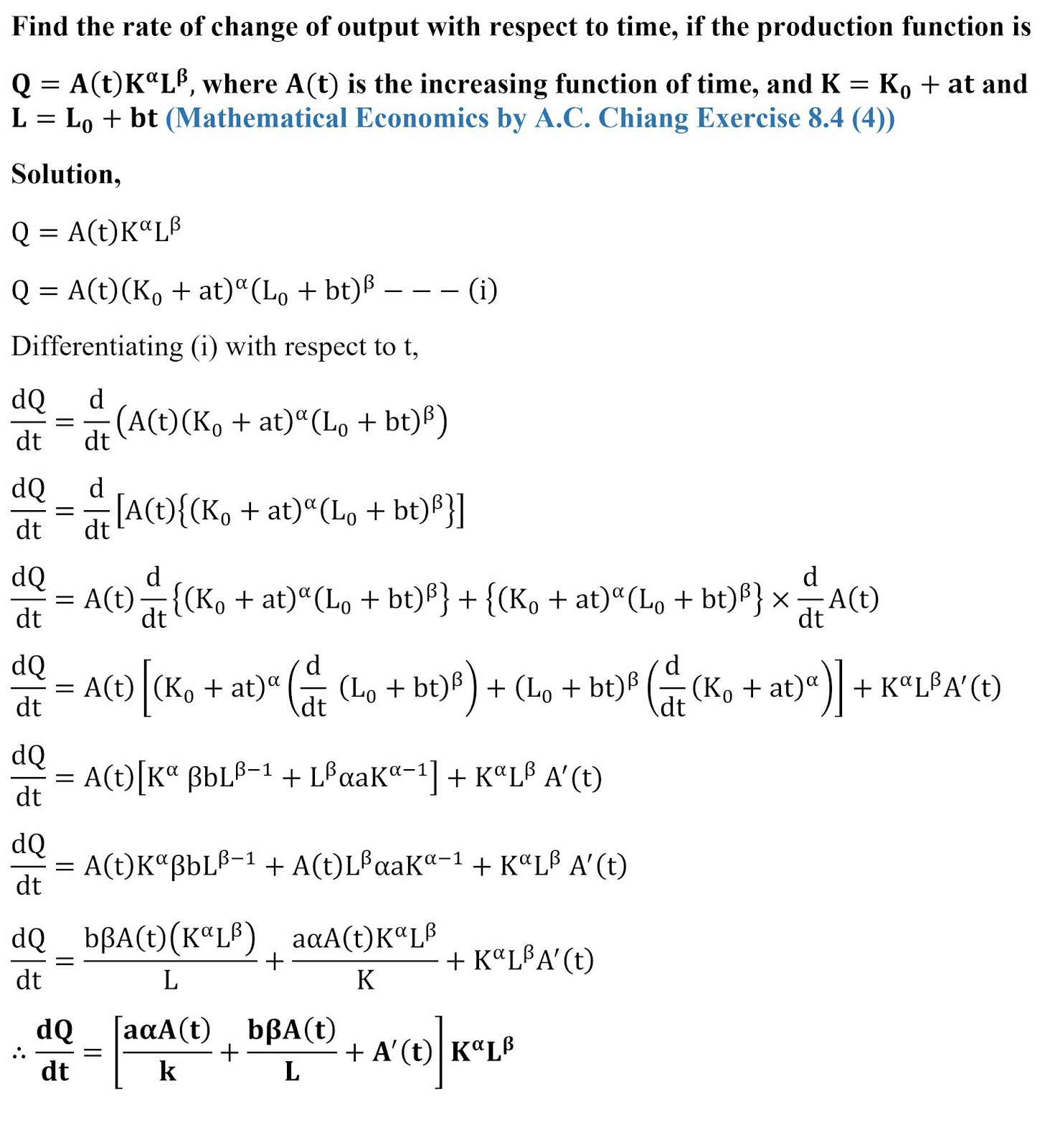# Q=A(t) K^α L^β, where A(t) is the increasing function of time (A.C. Chaing)

Find the rate of change of output with respect to time, if the production function is Q=A(t) K^α L^β, where A(t) is the increasing function of time, and K=K0+at and L=L0+bt (Mathematical Economics by A.C. Chiang Exercise 8.4 (4))